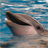# How to calculate the A2 parameter for the resolver

cancel
Showing results for
Did you mean:
SOLVED

## How to calculate the A2 parameter for the resolver

2,098 ViewsNXP TechSupport

Hello, all

I ask the question based on customer requirement

On the application note AN3943, there is an explanation to calculate parameters for a resolver with Mathlab (page 28).

With mathlab you can easily calculate paramter K1_scaled, K1_shift,K2scaled, K2_shift.

If you use ACLIB_AngleTrackObsvr from Aclib for 56800Ex, you have

K1_scaled, K1_shift,K2scaled, K2_shift and an extra parameter called A2_scaled, A2_shift.

Do you have a Mathlab method to calculate K1,K2, and A2 parameters. Regarding the A2 parameter definition (f16A2Scaled), pls refer to the 56800Ex_ACLIB.pdf

Thanks and best regards,

XiangJun Rong

Labels (1)
• ### General

1 Solution
1,517 ViewsNXP Employee

Ni hao XiangJun,

First, I'd recommend you use the latest library which can be downloaded at www.nxp.com/fslesl.

On the same link, you can find the latest documentation for AMCLIB on DSP56800E(X). You can consult the chapter describing AMCLIB_AngleTrackObsrv to learn the observer equations and the way they are scaled. The calculation of the parameters is described in the table dedicated to the algorithm's structure: AMCLIB_ANGLE_TRACK_OBSRV_T_F32;

The A2 gain parameter is taken from an equation and is given by the sampling time, speed and angle scales: Ts * speed_max / angle max. To use the maximum resolution bits of this parameter, it is good to keep it in the range from 0.5 to 1. To do this, you use its scale shift.

The K1 gain parameter is given by the sampling time, K1 constant and the scale of the speed: Ts * K1 / speed_max. It has the scale shift too.

The K2 gain parameter is given by the K2 constant, speed and angle scale: K2 * speed_max / angle_max. It has the scale shift too.

The value of K1 and K2 constants are given by your system.

Best Regards,

Jaroslav Musil

1,518 ViewsNXP Employee

Ni hao XiangJun,

First, I'd recommend you use the latest library which can be downloaded at www.nxp.com/fslesl.

On the same link, you can find the latest documentation for AMCLIB on DSP56800E(X). You can consult the chapter describing AMCLIB_AngleTrackObsrv to learn the observer equations and the way they are scaled. The calculation of the parameters is described in the table dedicated to the algorithm's structure: AMCLIB_ANGLE_TRACK_OBSRV_T_F32;

The A2 gain parameter is taken from an equation and is given by the sampling time, speed and angle scales: Ts * speed_max / angle max. To use the maximum resolution bits of this parameter, it is good to keep it in the range from 0.5 to 1. To do this, you use its scale shift.

The K1 gain parameter is given by the sampling time, K1 constant and the scale of the speed: Ts * K1 / speed_max. It has the scale shift too.

The K2 gain parameter is given by the K2 constant, speed and angle scale: K2 * speed_max / angle_max. It has the scale shift too.

The value of K1 and K2 constants are given by your system.

Best Regards,

Jaroslav Musil